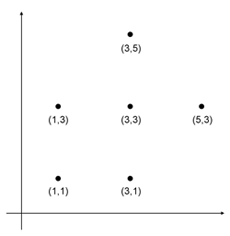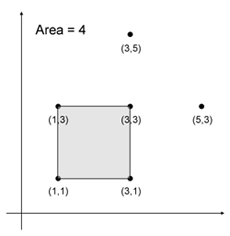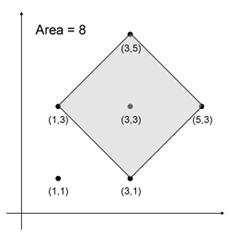# Square Lookup Easy

Time Limit: 1s

Given a set S of N points in Cartesian coordinate, find the largest square that can be formed by choosing four distinct points from S.### Input

The first line of input contains an integer T, the number of test cases follow.

Each case begins with an integer N (1 <= N <= 15). The next N following lines each consists of a pair of integers, the x and y coordinate of ith point of S in Cartesian coordinate respectively (-100 <= x, y <= 100).

### Output

For each case, output area of the largest square that can be formed by choosing four distinct points from S, rounded to two decimal points. Output "0.00" (without quote) if there are no squares can be formed.

Sample InputOutput for Sample Input
```2
3
1 1
5 5
1 5
6
1 1
3 1
3 3
1 3
5 3
3 5
```
```0.00
8.00```

Source: BNPC-HS 2007 Qualification Round
Modified for INC 2008 - Practice Session